# Practice Exercises for Multiplying and Dividing Decimals

Directions: Read each question below. You may use paper and pencil to help you solve these problems. Select your answer by clicking on its button. Feedback to your answer is provided in the RESULTS BOX. If you make a mistake, choose a different button.

 1 Choose the best estimate for 8 x 7.29.
 5.6       56.0       560.       5,600.       RESULTS BOX:
 2 Choose the best estimate for 37.7 x 0.4.
 1,600.       160.       16.       None of the above       RESULTS BOX:
 3 Which of the following is the correct product when you multiply 6.24 by 1000?
 6.24       62.4       624.       6,240.       RESULTS BOX:
 4 Which of the following is the correct product when you multiply 2.75 by 0.5?
 1,375.       13.75       1.375       None of the above.       RESULTS BOX:
 5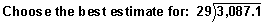100       10       1       0.1       RESULTS BOX:
 6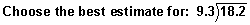200       20       2       All of the above.       RESULTS BOX:
 7 Which of the following is the correct answer when you divide the numbers below, and then round the quotient to the nearest tenth?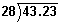1.5       1.5439285       15.43       15.439285       RESULTS BOX:
 8 Which of the following is the correct answer when you divide the numbers below, and then round the quotient to the nearest cent?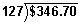\$2,730.00       \$273.00       \$2.73       None of the above.       RESULTS BOX:
 9 Which of the following is the quotient when you divide the numbers below?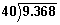0.2342       2.342       23.42       All of the above.       RESULTS BOX:
 10 Which of the following is the quotient when you divide the numbers below?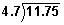0.025       0.25       2.5       None of the above.       RESULTS BOX: